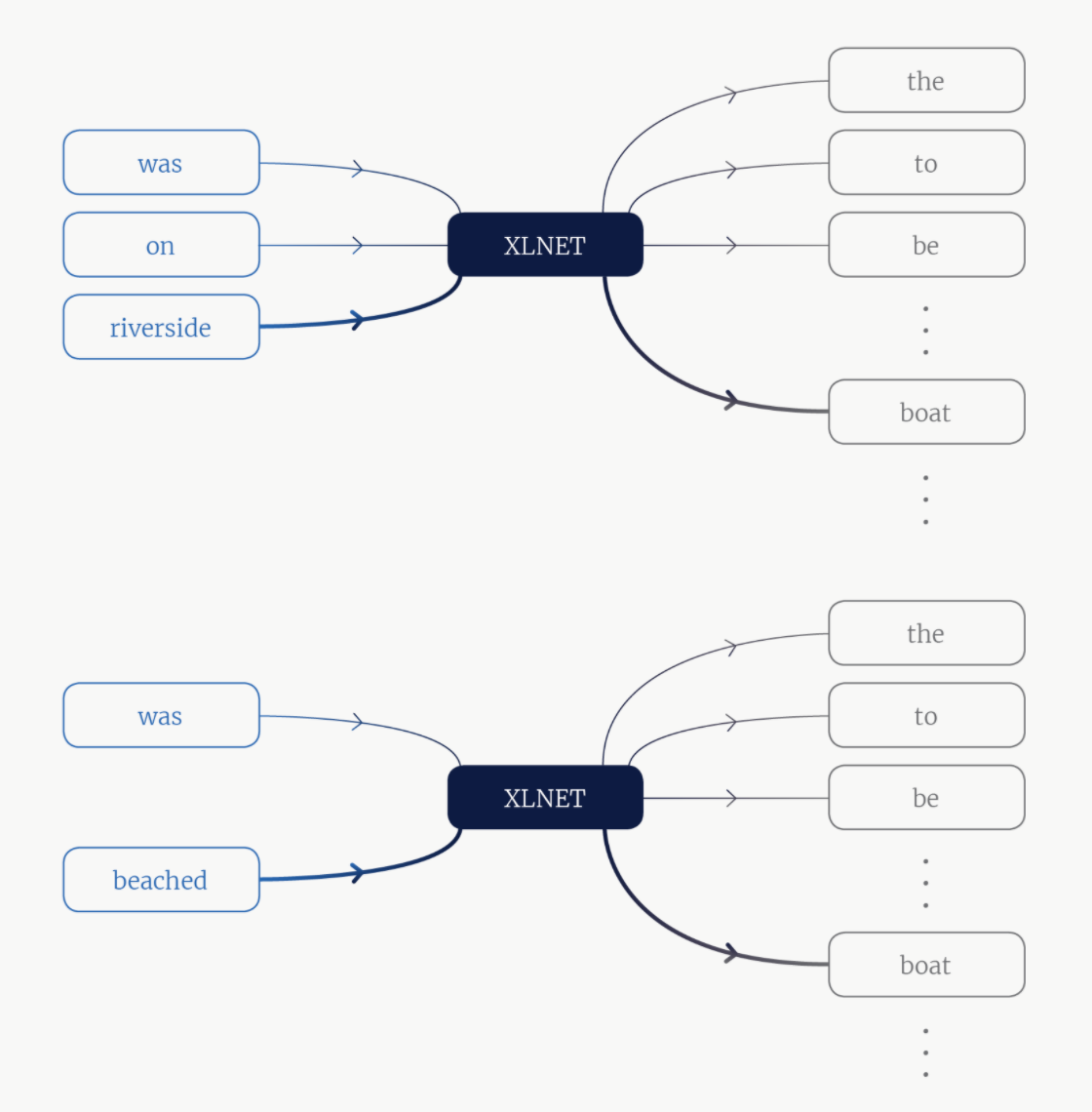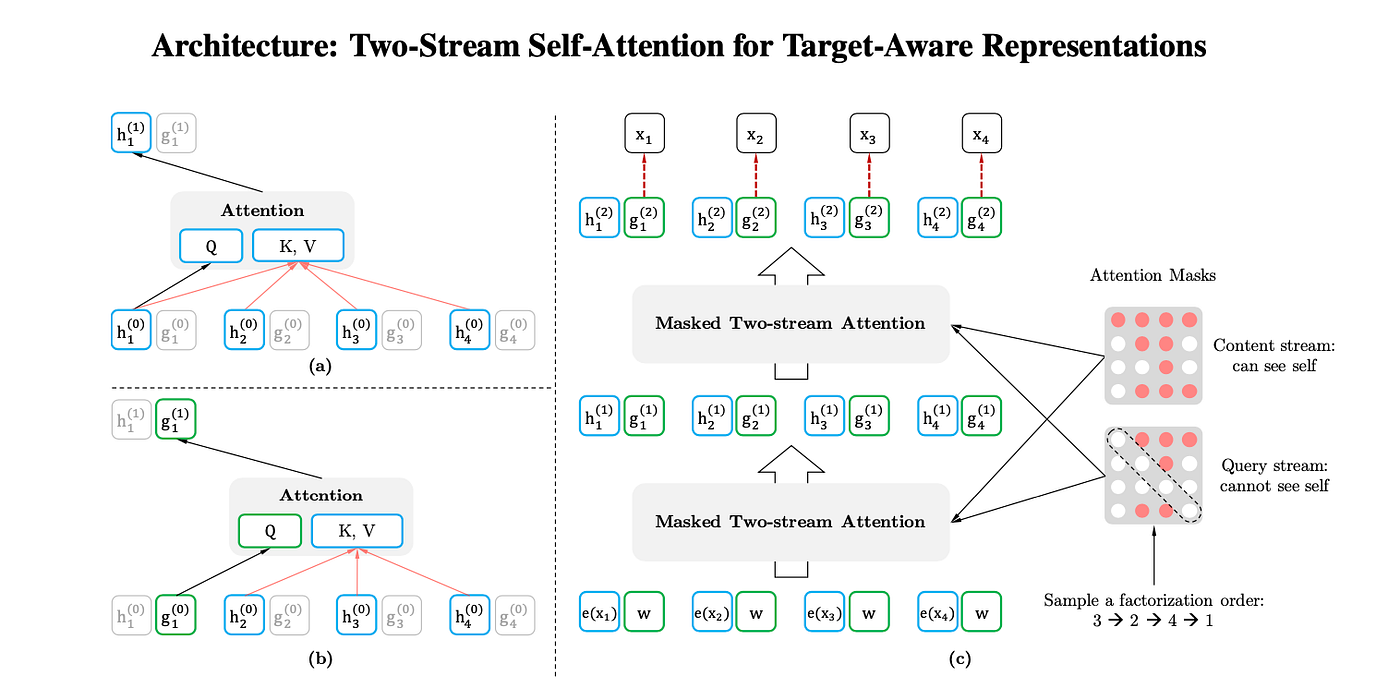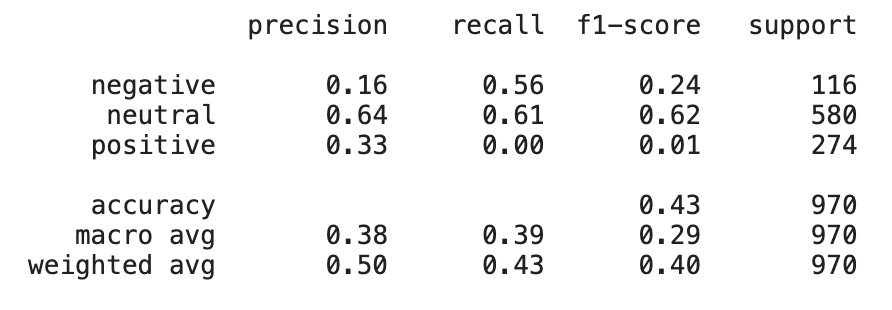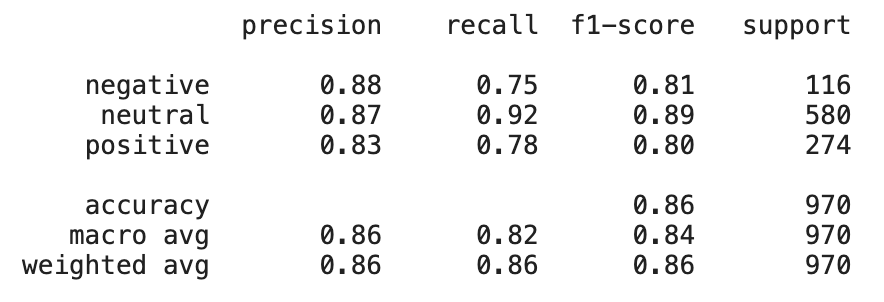# Use NLP to Take on Wall StreetXLNet model — must calculate that boat is likely token for many different contexts drawn from the sequencea): content stream attention b): query stream attention c): overview of permutation language modeling training with two-stream attentionConfusion Matrix of XLNet model without retrainingConfusion Matrix of XLNet model after fine-tuning
• It gets the stock price information and financial news headlines for the stocks in the Dow Jones Index.
• First, it pulls the stock price information for the 30 stocks in the index using Yfinance’s API. That data is stored in a data frame and a CSV file is created.
• Second, we use Finnhub’s API to get news headlines relevant to the stocks in the Dow Jones Index and those headlines are stored in another data frame and a CSV file is created for them.
• Third, the stock price CSV and news headlines CSV file are both uploaded to Google Cloud storage.
`# Script 1 - gets news headlines and stock price data# Import necessary packagesimport yfinance as yfimport jsonimport datetimeimport requestsimport pandas as pdimport pytz# Set the start and end datestart_date = datetime.datetime.now(pytz.timezone('US/Pacific')).strftime('%Y-%m-%d')end_date = (datetime.datetime.now(pytz.timezone('US/Pacific')) + datetime.timedelta(days=1)).strftime('%Y-%m-%d')# Opening JSON file - it has all the stock tickersf = open("config.json", )# Returns JSON object as a dictionaryconfig = json.loads(f.read())# Function to get stock pricesdef get_stock_data(stockticker, startdate, enddate):    data = yf.download(stockticker, startdate, enddate)    data['name'] = stockticker    return data# Function to get news headlinesdef get_news_data(stockticker, startdate, enddate):    url = f"https://finnhub.io/api/v1/company-news?symbol={stockticker}&from={startdate}&to={enddate}&token=c2mnsqqad3idu4aicnrg"    r = requests.get(url)    response = r.json()    if not response:        return pd.DataFrame(index=['datetime', 'headline', 'related', 'source'])    r2 = pd.DataFrame(response)    df = r2[['datetime', 'headline', 'related', 'source']]    return df# Get stock information about multiple stocksstock_data_list = []for ticker in config["stockticker"].split():    tmp = get_stock_data(ticker, start_date, end_date)    if not tmp.empty:        stock_data_list.append(tmp)stock_data = pd.concat(stock_data_list)# Get news information about multiple stocksnews_data_list = []for ticker in config["stockticker"].split():    tmp = get_news_data(ticker, start_date, end_date)    if not tmp.empty:        news_data_list.append(tmp)news_data = pd.concat(news_data_list)# Upload CSV files to Google cloudfrom google.cloud import storageclient = storage.Client.from_service_account_json(json_credentials_path='yourfile.json')bucket = client.get_bucket('yourbucket1')object_name_in_gcs_bucket = bucket.blob('stock_data.csv')df = pd.DataFrame(data=stock_data).to_csv(encoding="UTF-8")object_name_in_gcs_bucket.upload_from_string(data=df)object_name_in_gcs_bucket = bucket.blob('news_data.csv')df = pd.DataFrame(data=news_data).to_csv(encoding="UTF-8")object_name_in_gcs_bucket.upload_from_string(data=df)`
• It first pulls the news headlines CSV file from Google Cloud.
• Then, we load Google’s XLNet model with our pre-trained weights from our checkpoint file that we created when we fine-tuned the model for sentiment analysis based on the Financial Phrase-bank dataset. Then we do some data-preprocessing steps because the model takes input in a certain way (tokenize the inputs) and then the predict_sentiment function determines the polarity of a news headline.
• Then, the second stock price CSV file is pulled from Google Cloud. The two data frames are concatenated and a completed processed CSV file is uploaded to another bucket on Google Cloud.
• Then, on the Google Cloud Platform we used the Big Query database and linked it to Google storage buckets so the completed processed file was uploading data to the database.
`# Model Inferenceimport pandas as pdimport torchimport torch.nn.functional as Ffrom transformers import XLNetModel, XLNetTokenizer, XLNetForSequenceClassificationfrom keras.preprocessing.sequence import pad_sequencesimport timeimport io# Get CSV files from Google Cloudfrom google.cloud import storageclient = storage.Client.from_service_account_json(json_credentials_path='yourfile.json')bucket = client.bucket('yourbucket1')blob = bucket.blob('news_data.csv')blob.download_to_filename('data.csv')df = pd.read_csv('data.csv')# Load the XLNET model and pre-trained weightsmodel = XLNetForSequenceClassification.from_pretrained("xlnet-base-cased", num_labels=3)model.load_state_dict(torch.load("model_with_retraining.ckpt", map_location=torch.device('cpu')))# keep map_location# model.cuda()# Prediction function to determine sentiment of news headlinesdef predict_sentiment(text):    review_text = text    encoded_review = tokenizer.encode_plus(    review_text,    max_length=MAX_LEN,    add_special_tokens=True,    return_token_type_ids=False,    pad_to_max_length=False,    return_attention_mask=True,    return_tensors='pt',    )    input_ids = pad_sequences(encoded_review['input_ids'], maxlen=MAX_LEN, dtype=torch.Tensor ,truncating="post",padding="post")    input_ids = input_ids.astype(dtype = 'int64')    input_ids = torch.tensor(input_ids)    attention_mask = pad_sequences(encoded_review['attention_mask'], maxlen=MAX_LEN, dtype=torch.Tensor ,truncating="post",padding="post")    attention_mask = attention_mask.astype(dtype = 'int64')    attention_mask = torch.tensor(attention_mask)    input_ids = input_ids.reshape(1,128).to(device)    attention_mask = attention_mask.to(device)    outputs = model(input_ids=input_ids, attention_mask=attention_mask)    outputs = outputs.cpu().detach()    probs = F.softmax(outputs, dim=-1).cpu().detach().numpy().tolist()    _, prediction = torch.max(outputs, dim =-1)    target_names = ['negative', 'neutral', 'positive']    return probs, target_names[prediction]device = torch.device("cuda" if torch.cuda.is_available() else "cpu")tokenizer = XLNetTokenizer.from_pretrained('xlnet-base-cased')MAX_LEN = 128probs_list = []prediction_list = []for sentence in df['headline']:    probs, prediction = predict_sentiment(sentence)    probs_list.append(probs)    prediction_list.append(prediction)probs_df = pd.DataFrame(probs_list)probs_df.columns = ['negative', 'neutral', 'positive']prediction_df = pd.DataFrame(prediction_list)prediction_df.columns = ['Sentiment']# Classified news headlinesfinal_df = pd.concat([df,probs_df,prediction_df], axis=1)final_df["datetime"] = pd.to_datetime(final_df["datetime"], unit='s').dt.strftime('%Y-%m-%d')final_df = final_df.rename(columns={"datetime":"Date"})final_df = final_df.rename(columns={"related":"name"})# Get Stock Price CSV and concatenate stock price and processed headlines into one CSV fileblob = bucket.blob('stock_data.csv')blob.download_to_filename('stock1.csv')hist = pd.read_csv('stock1.csv')pd.set_option('display.max_columns', None)complete_df = pd.merge(final_df, hist, how='left', on=['Date', 'name'])# Posting data to Google cloudbucket = client.get_bucket('yourbucket2')object_name_in_gcs_bucket = bucket.blob('complete_df_'+ time.strftime('%Y%m%d')+'.csv')df = pd.DataFrame(data=complete_df).to_csv(encoding="UTF-8")object_name_in_gcs_bucket.upload_from_string(data=df)`
`# Load Libraries from google.cloud import bigqueryimport json# Function to return data from Big Query Database def query_stackoverflow(stock):    client = bigquery.Client.from_service_account_json(json_credentials_path='yourfile.json')    query_job = client.query(        """        SELECT * FROM `sunlit-inquiry-319400.ucsdcapstonedataset.StockData` WHERE name = '"""+stock+"""' LIMIT 1000        """    )    results = query_job.result()    htmlmsg = "<html><body><table border=\"1\" style=\"border-collapse:collapse;\"><tr><td>Date</td><td>Headline</td><td>Name</td><td>Sentiment</td><td>Close</td><td>Volume</td></tr>"    for row in results:        htmlmsg += "<tr><td>" + str(row) + "</td><td>" + str(row) + "</td><td>" + str(row) + "</td><td>" + str(row) + "</td><td>" + str(row) + "</td><td>" + str(row)+ "</td></tr>"    htmlmsg += "</table></body></html>"    return htmlmsgfrom flask import Flask, render_template, request, jsonifyapp = Flask(__name__)# Flask home page @app.route('/')def home():   return render_template('index.html')@app.route('/songs', methods=['POST', 'GET'])def get_info():    stock = request.form.get("stock")    print(stock)    return query_stackoverflow(stock)if __name__ == '__main__':  app.run(host='0.0.0.0')`
1. Create a docker file which is the blueprint for building images
2. Build a docker image which the blueprint for the container
3. Run the container to see the output of the packaged product